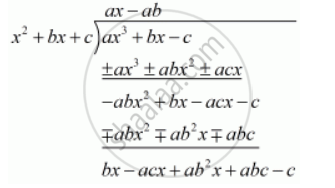Advertisement Remove all ads

# If Q.No. 14, C = - Mathematics

MCQ

If Q.No. 14, c =

#### Options

•  b

• 2b

• 2b2

• −2b

Advertisement Remove all ads

#### Solution

We have to find the value of c

Given  f(x)= ax^3 +bx -c is divisible by the polynomial g (x )= x^2 + bx + cWe must have

bx - acx +ab^2x + abc -c = 0  for all x

 x (b - ac + ab^2) + c (ab-1)= 0..........(1)

c (ab-1)=0

Since  c  ≠ 0, so

ab - 1 = 0

ab = 1

Now in the equation (1) the condition is true for all x. So put = 1 and also we have ab = 1

Therefore we have

b - ac + ab^2 = 0

b +ab^2 -ac =0

b(a +ab)-ac = 0

Substituting a= 1/band  ab =1 we get,

b(1 + 1) - 1/b xxc = 0

2b -1/b xxc =0

- 1/b xxc = -2b

cancel(-)1/bxx cancel(-) 2b

 c = 2b =b/1

c = 2b ^2

Hence, the correct alternative is (c)

Is there an error in this question or solution?
Advertisement Remove all ads

#### APPEARS IN

RD Sharma Class 10 Maths
Chapter 2 Polynomials
Q 15 | Page 63
Advertisement Remove all ads

#### Video TutorialsVIEW ALL 

Advertisement Remove all ads
Share
Notifications

View all notifications

Forgot password?